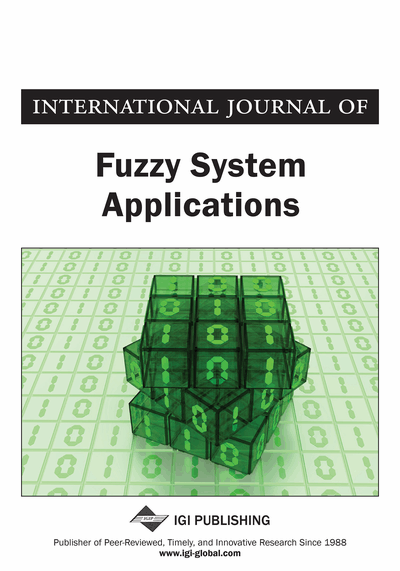# Vague Correlation Coefficient of Interval Vague Sets

P. John Robinson, E. C. Henry Amirtharaj
DOI: 10.4018/ijfsa.2012010102
OnDemand:
(Individual Articles)
Available
\$37.50
No Current Special Offers

## Abstract

Various attempts have been made by researchers on the study of vagueness of data through intuitionistic fuzzy sets and vague sets, and also it was shown that vague sets are intuitionistic fuzzy sets. But there are algebraic and graphical differences between vague sets and intuitionistic fuzzy sets. In this paper an attempt is made to define the correlation coefficient of interval vague sets lying in the interval [0, 1], and a new method for computing the correlation coefficient of interval vague sets lying in the interval [-1, 1] using a-cuts over the vague degrees through statistical confidence intervals is presented by an example. The new method proposed in this paper produces a correlation coefficient in the form of an interval.
Article Preview
Top

## Introduction

Correlation coefficient of fuzzy sets, interval-valued fuzzy sets (IFS), intuitionistic fuzzy sets and interval-valued intuitionistic fuzzy sets (IVIFS) are already in the literature. Various attempts are made by researchers in the recent days in defining the correlation coefficient of intuitionistic fuzzy sets and interval-valued intuitionistic fuzzy sets. Atanassov (1986, 1989) proposed the Intuitionistic fuzzy set theory. Gau and Buehrer (1994) proposed the concept of vague sets. Bustince and Burillo (1996) proposed that vague sets are indeed intuitionistic fuzzy sets and they unified vague sets and intuitionistic fuzzy sets. Since vague sets deal with truth membership value, false membership value and the vague degree, and has more ability to deal with uncertain information than the traditional fuzzy sets (Lu & Ng 2005, 2009), many researchers pay attention on vague set theory. Atanassov and Gargov (1989) extended the intuition vague sets and proposed the concept of interval intuition vague sets, also named as interval vague sets. Interval vague sets can better express vagueness and uncertainty. Atanassov (1994) has focused on the operational rules of the interval vague sets. Bustince and Burillo (1995) and Hong (1998) have focused on the correlation degree of interval valued intuitionistic fuzzy sets. Park et al. (2009) have also worked on the correlation coefficient of interval valued intuitionistic fuzzy sets and applied in multiple-attribute group decision making problems. Kao and Liu (2002) introduced fuzzy measures for the correlation coefficient of fuzzy numbers lying in the interval [-1, 1]. Buckley (2004), using the approach of fuzzy probabilities, defined the fuzzy correlation coefficient. Zeng and Li (2007) focused on the probability spaces to define a new kind of correlation for intuitionistic fuzzy sets. Gerstenkon and Manko (1991) defined the correlation of intuitionistic fuzzy sets. Hong and Hwang (1995) defined it on probability spaces. Hung and Wu (2002) introduced the concepts of positively and negatively correlated results based on the concept of centroid for intuitionistic fuzzy sets lying in the interval [-1, 1]. Hong (1998) studied the correlation coefficient of interval-valued intuitionistic fuzzy sets in probability spaces. Mitchell (2004) adopted a statistical view point to interpret intuitionistic fuzzy sets as an ensemble of ordinary fuzzy set, and defined the correlation coefficient of intuitionistic fuzzy sets by using the correlation coefficient of two ordinary fuzzy sets and a mean aggregation function.

At present, the researches of vague set are focused on the operation rules of the interval Vague set, correlation degree (Bustince & Burillo, 1995; Hong, 1998; Robinson & Amirtharaj, 2011), and topological structures. This paper focuses on interval vague sets. Gau and Buehrer (1993), Xu and Chen (2007), Li and Rao (2001), and Piede Liu (2009) have presented in detail the essential operations of interval vague sets. Interval vague set is one of the higher order fuzzy sets that are now in the literature and are being applied into many specialized application domains. The notions of truth membership function, false membership function and uncertainty function in interval vague sets can describe the objective world more realistic and practical. Interval vague sets can reflect people’s understanding comprehensively in three aspects: Support degree, Negative degree and Uncertainty degree. Combining the interval-valued fuzzy sets and vague sets, Ma et al. (2001) introduced the concept of interval valued vague sets.

## Complete Article List

Search this Journal:
Reset
Volume 12: 1 Issue (2023)
Volume 11: 4 Issues (2022)
Volume 10: 4 Issues (2021)
Volume 9: 4 Issues (2020)
Volume 8: 4 Issues (2019)
Volume 7: 4 Issues (2018)
Volume 6: 4 Issues (2017)
Volume 5: 4 Issues (2016)
Volume 4: 4 Issues (2015)
Volume 3: 4 Issues (2013)
Volume 2: 4 Issues (2012)
Volume 1: 4 Issues (2011)
View Complete Journal Contents Listing